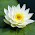## 20.7.13

### C++ : Object and Class with a traditional BOX example

A class definition starts with the keyword class followed by the class name; and the class body, enclosed by a pair of curly braces. A class definition must be followed either by a semicolon or a list of declarations. For example we defined the Box data type using the keyword class as follows:
```
```
```class Box
{
public:
double length;   // Length of a box
double breadth;  // Breadth of a box
double height;   // Height of a box
};```

The keyword public determines the access attributes of the members of the class that follow it.
A public member can be accessed from outside the class anywhere within the scope of the class object.
You can also specify the members of a class as private or protected which we will discuss in a sub-section.

When you define a class, you define a blueprint for a data type. This doesn't actually define any data, but it does define what the class name means, that is, what an object of the class will consist of and what operations can be performed on such an object.

A class provides the blueprints for objects, so basically an object is created from a class. We declare objects of a class with exactly the same sort of declaration that we declare variables of basic types. Following statements declare two objects of class Box:
```Box Box1;          // Declare Box1 of type Box
Box Box2;          // Declare Box2 of type Box```
Both of the objects Box1 and Box2 will have their own copy of data members.

## The Access method between Object and Class:

The public data members of objects of a class can be accessed using the direct member access operator (.). Let us try following example to make the things clear:

```#include <iostream>

using namespace std;

class Box
{
public:
double length;   // Length of a box
double breadth;  // Breadth of a box
double height;   // Height of a box
};

int main( )
{
Box Box1;        // Declare Box1 of type Box
Box Box2;        // Declare Box2 of type Box
double volume = 0.0;     // Store the volume of a box here

// box 1 specification
Box1.height = 5.0;
Box1.length = 6.0;
Box1.breadth = 7.0;

// box 2 specification
Box2.height = 10.0;
Box2.length = 12.0;
Box2.breadth = 13.0;
// volume of box 1
volume = Box1.height * Box1.length * Box1.breadth;
cout << "Volume of Box1 : " << volume <<endl;

// volume of box 2
volume = Box2.height * Box2.length * Box2.breadth;
cout << "Volume of Box2 : " << volume <<endl;
return 0;
}```
When the above code is compiled and executed, it produces following result:
```Volume of Box1 : 210
Volume of Box2 : 1560```

It is important to note that private and protected members can not be accessed directly using direct member access operator (.). We will learn how private and protected members can be accessed.

### Read Class and Object with Constructor and Destructor  with the Exercise of Area of a CUBE :

#### 2 comments:

1.using C++
#include

using namespace std;

class Box
{
public:
double width;
double length;
double height;
Box();
Box(double width, double height, double length);
~Box();
};
Box::Box()
{
cout << "It will be calculate the area of box.\n";
cout << "Enter the value of width of your box --: ";
cin >> width;
cout << "Enter the value of length of your box --: ";
cin >> length;
cout << "Enter the value of height of your box --: ";
cin >> height;

cout << "The area of the box is --: " << width*height*length << "\n";
}

Box::Box(double width, double height, double length)
{
cout << "The area of the box is --: " << width*height*length << "\n";
}

Box::~Box()
{
cout << "[+]Memory released[+]";
}

int main()
{
Box objOne;

double width;
double length;
double height;

cout << "This will be also calculate the area of box -- \n";
cout << "Enter the value of width of your box --: ";
cin >> width;
cout << "Enter the value of length of your box --: ";
cin >> length;
cout << "Enter the value of height of your box --: ";
cin >> height;

Box ObjTwo(width, height, length);

return 0;
}

2.Marvelous and fascinating article. Incredible things you've generally imparted to us. Much obliged. Simply keep making this kind out of the post.
C plus plus Courses in Chennai

Comment Here